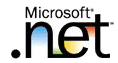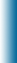Welcome to Mike95.com
 Home Features C++ Library Source Code: COM / ASP Tutorials: Sample Work Internet Based Visual C++ / MFC Java Personal
C++ Templates
Static Members and Variables

Each template class or function generated from a template has its own copies of any static variables or members.

Each instantiation of a function template has it's own copy of any static variables defined within the scope of the function. For example,

```template <class T>
class X
{
public:
static T s;
};

int main()
{
X<int> xi;
X<char*> xc;
}
```

Here X<int> has a static data member s of type int and X<char*> has a static data member s of type char*. Static members are defined as follows.

```#include <iostream>
using namespace std;

template <class T>
class X
{
public:
static T s;
};

template <class T> T X<T>::s = 0;  //or the type being used.
template <> int X<int>::s = 3;
template <> char* X<char*>::s = "Hello";

int main()
{
X<int> xi;
cout << "xi.s = " << xi.s << endl;

X<char*> xc;
cout << "xc.s = " << xc.s << endl;

return 0;
}
```

### Program Output

```xi.s = 3
xc.s = Hello
```

Each instantiation of a function template has it's own copy of the static variable. For example,

```#include <iostream>
using namespace std ;

template <class T>
void f(T t)
{
static T s  = 0;
s = t;
cout << "s = " << s << endl;
}

int main()
{
f(10);
f("Hello");

return 0;
}
```

### Program Output

```s = 10
s = Hello
```
Here f<int>(int) has a static variable s of type int, and f<char*>(char*) has a static variable s of type char*.
 (c)2023 Mike95.com / Site Disclaimer